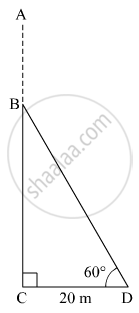Share

# A Storm Broke a Tree and the Treetop Rested 20 M from the Base of the Tree, Making an Angle of 60° with the Horizontal. Find the Height of the Tree. - Geometry

ConceptHeights and Distances

#### Question

A storm broke a tree and the treetop rested 20 m from the base of the tree, making an angle of 60° with the horizontal. Find the height of the tree.

#### SolutionLet AC be the original height of the tree. Suppose BD be the broken part of the tree which is rested at D from the base of the tree.
Here, CD = 20 m and ∠BDC = 60º.
In right ∆BCD,
$\tan60^\circ = \frac{BC}{CD}$
$\Rightarrow \sqrt{3} = \frac{BC}{20}$
$\Rightarrow BC = 20\sqrt{3} m . . . . . \left( 1 \right)$
Also,
$\cos60^\circ = \frac{CD}{BD}$
$\Rightarrow \frac{1}{2} = \frac{20}{BD}$
$\Rightarrow BD = 40 m . . . . . \left( 2 \right)$
∴ Height of the tree = AB + BC = BD + BC =
$\left( 40 + 20\sqrt{3} \right) m$               [Using (1) and (2)]
Thus, the height of the tree is $\left( 40 + 20\sqrt{3} \right) m$

Is there an error in this question or solution?

#### APPEARS IN

Balbharati Solution for Balbharati Class 10 Mathematics 2 Geometry (2018 to Current)
Chapter 6: Trigonometry
Practice set 6.2 | Q: 5 | Page no. 137

#### Video TutorialsVIEW ALL 

Solution A Storm Broke a Tree and the Treetop Rested 20 M from the Base of the Tree, Making an Angle of 60° with the Horizontal. Find the Height of the Tree. Concept: Heights and Distances.
S